# Graph Signal Processing for Machine Learning A Review and New Perspectives - Part 3

26 de Oct de 2021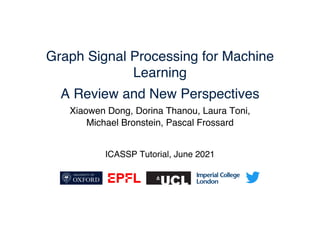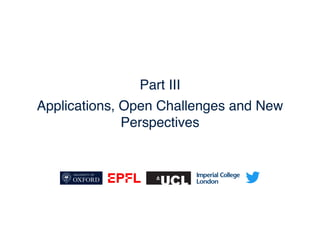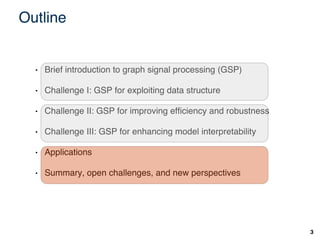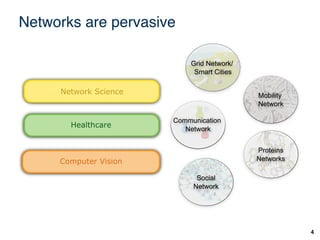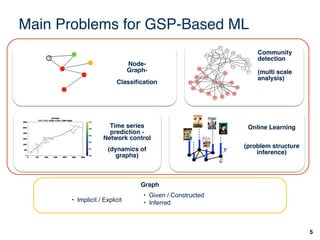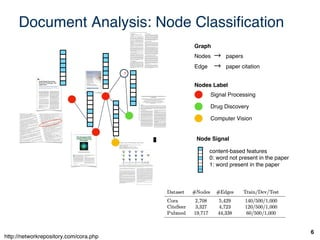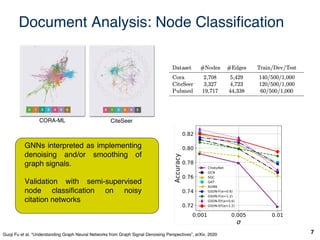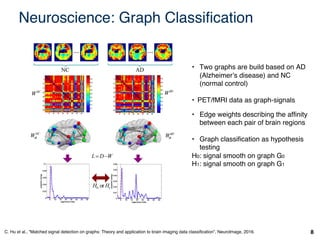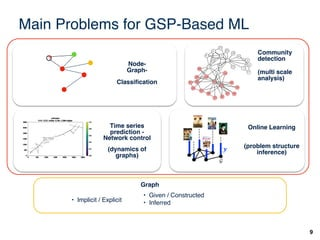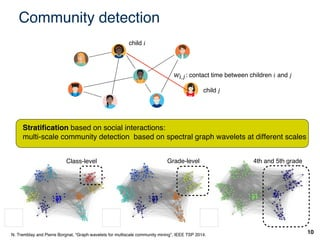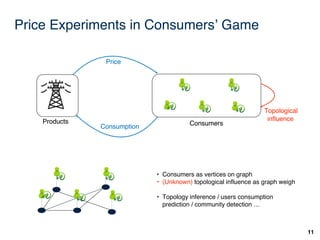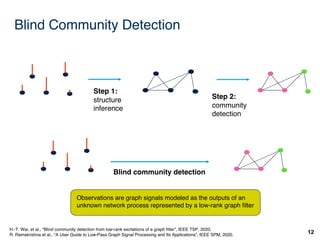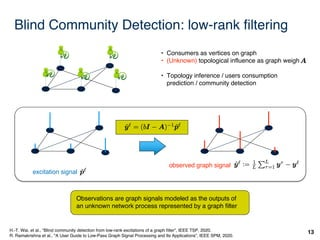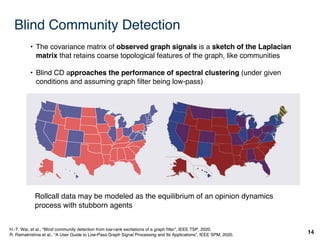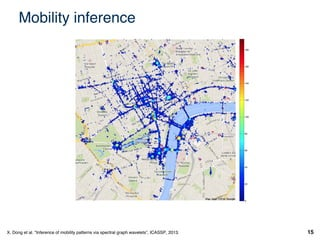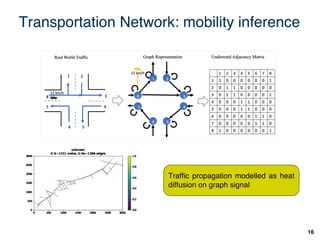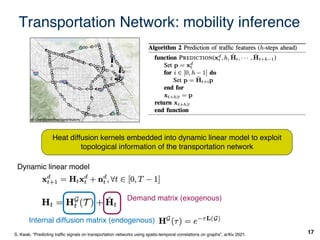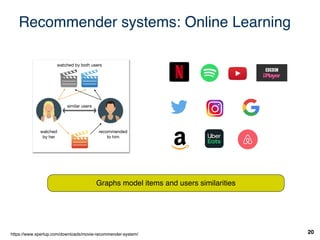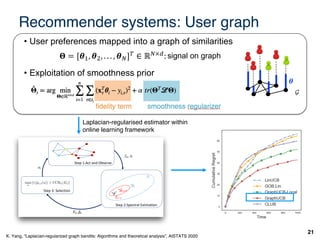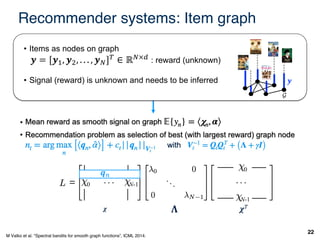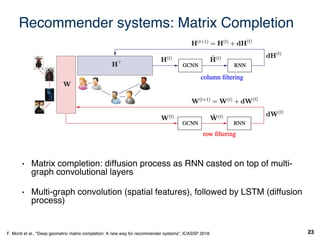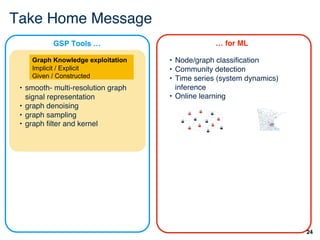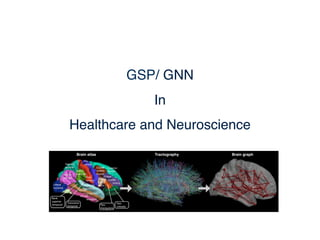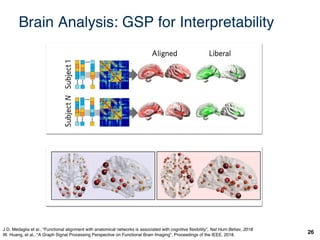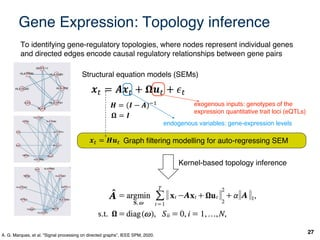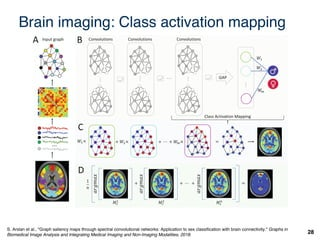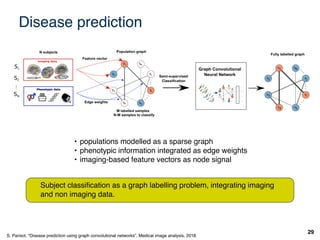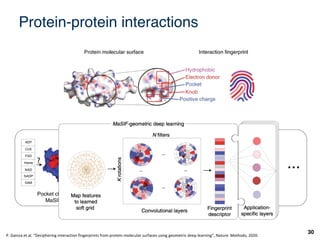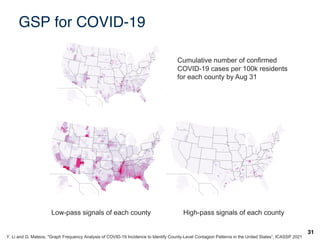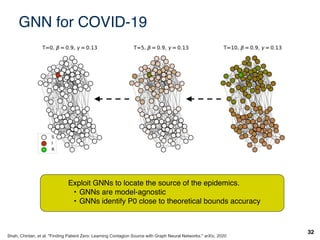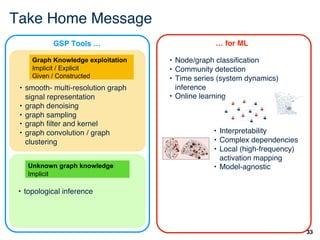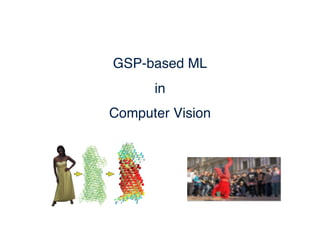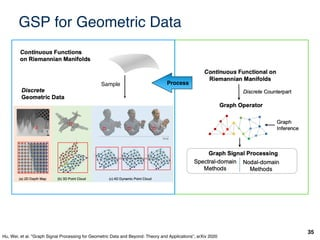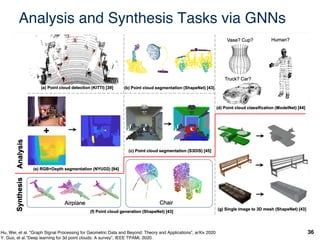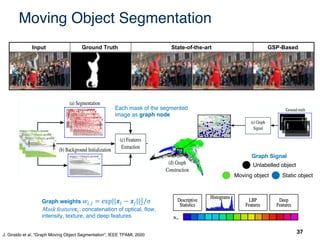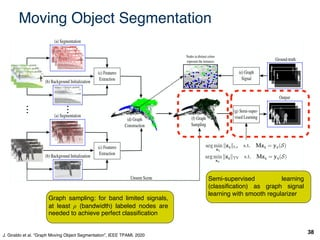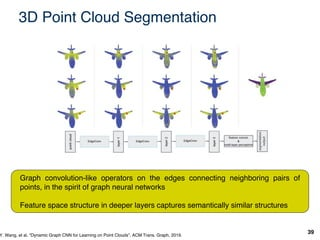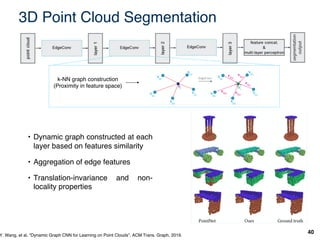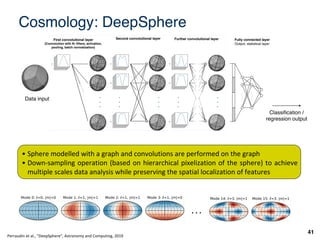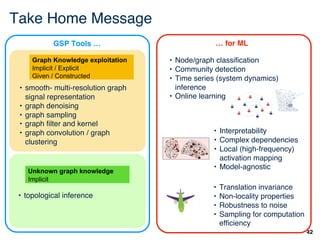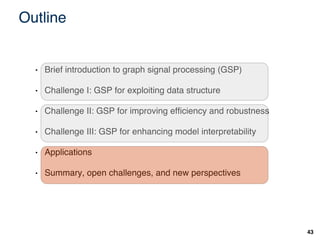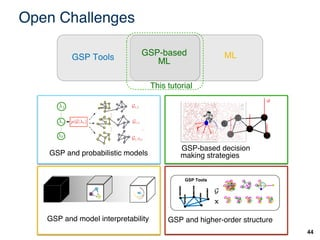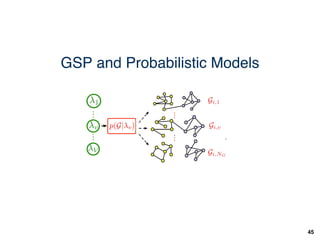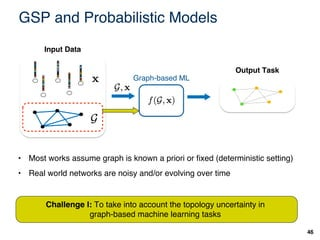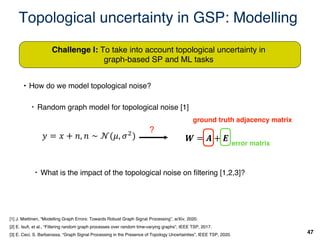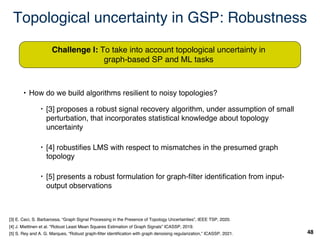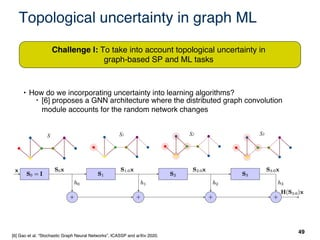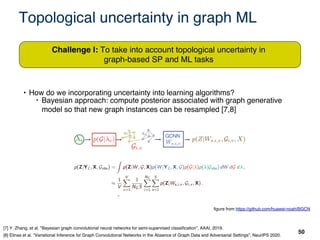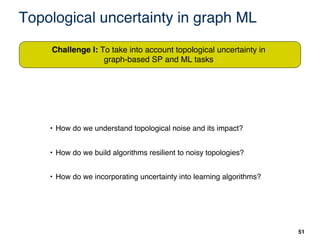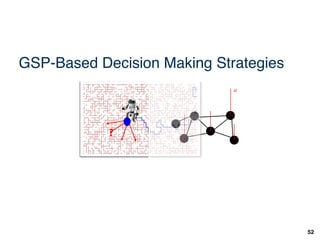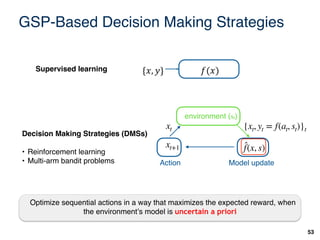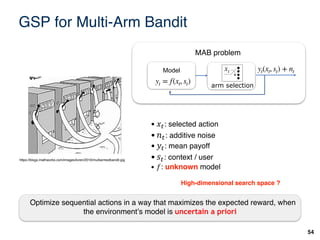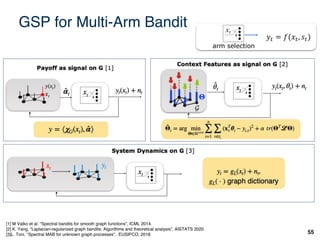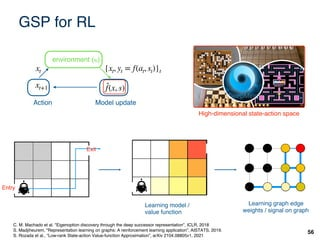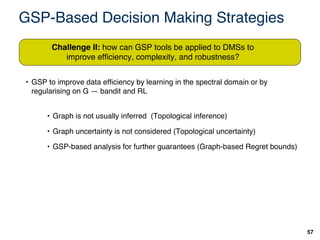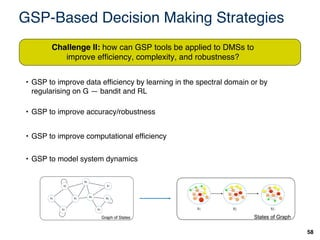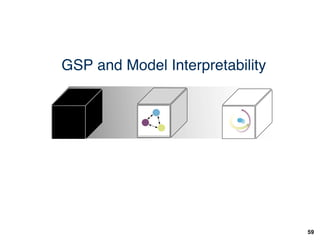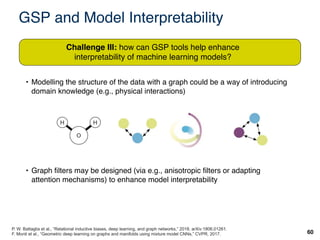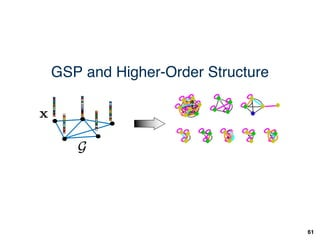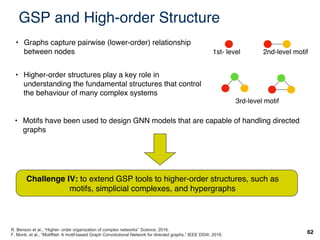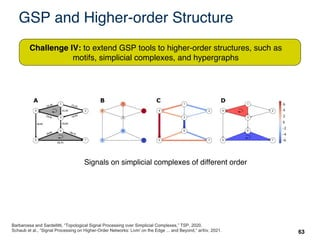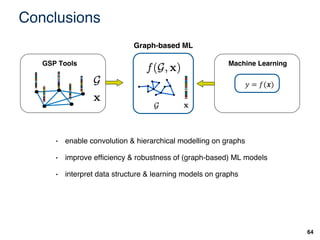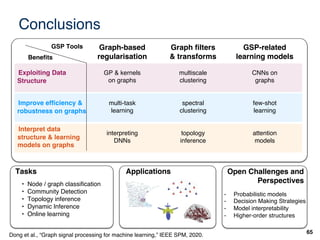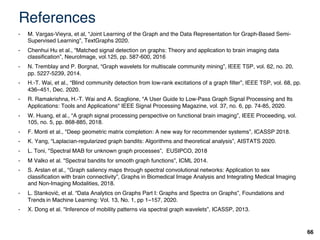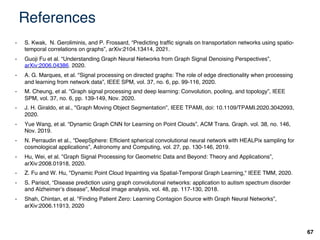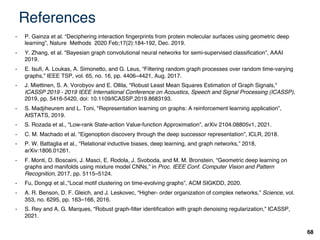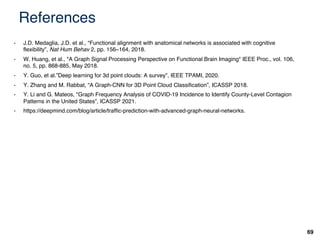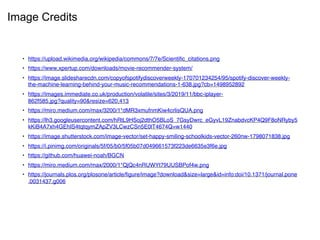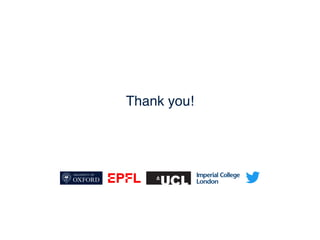1 de 69

### Graph Signal Processing for Machine Learning A Review and New Perspectives - Part 3

• 1. Graph Signal Processing for Machine Learning A Review and New Perspectives ICASSP Tutorial, June 2021 Xiaowen Dong, Dorina Thanou, Laura Toni, Michael Bronstein, Pascal Frossard
• 2. Part III Applications, Open Challenges and New Perspectives
• 3. Outline 3 • Brief introduction to graph signal processing (GSP) • Challenge I: GSP for exploiting data structure • Challenge II: GSP for improving efficiency and robustness • Challenge III: GSP for enhancing model interpretability • Applications • Summary, open challenges, and new perspectives
• 4. Networks are pervasive 4 Communication Network Grid Network/ Smart Cities Mobility Network Proteins Networks Social Network Healthcare Computer Vision Network Science
• 5. Main Problems for GSP-Based ML 5 Node- Graph- Classification Community detection (multi scale analysis) (dynamics of graphs) Time series prediction - Network control Online Learning (problem structure inference) 𝒚 • Implicit / Explicit • Given / Constructed • Inferred Graph ?
• 6. Document Analysis: Node Classification 6 Signal Processing Drug Discovery Computer Vision Nodes Label Graph Nodes → papers Edge → paper citation Node Signal content-based features 0: word not present in the paper 1: word present in the paper ? http://networkrepository.com/cora.php
• 7. Document Analysis: Node Classification 7 CORA-ML CiteSeer GNNs interpreted as implementing denoising and/or smoothing of graph signals. Validation with semi-supervised node classification on noisy citation networks Guoji Fu et al. “Understanding Graph Neural Networks from Graph Signal Denoising Perspectives”, arXiv, 2020
• 8. Neuroscience: Graph Classification 8 C. Hu et al., “Matched signal detection on graphs: Theory and application to brain imaging data classification”, NeuroImage, 2016. • Two graphs are build based on AD (Alzheimer’s disease) and NC (normal control) • PET/fMRI data as graph-signals • Edge weights describing the affinity between each pair of brain regions • Graph classification as hypothesis testing H0: signal smooth on graph G0 H1: signal smooth on graph G1
• 9. Main Problems for GSP-Based ML 9 Node- Graph- Classification Community detection (multi scale analysis) (dynamics of graphs) Time series prediction - Network control Online Learning (problem structure inference) 𝒚 • Implicit / Explicit ? ? • Given / Constructed • Inferred Graph
• 10. Community detection 10 N. Tremblay and Pierre Borgnat, “Graph wavelets for multiscale community mining”, IEEE TSP 2014. 𝑤!,#: contact time between children 𝑖 and 𝑗 child 𝑖 child 𝑗 Class-level Grade-level 4th and 5th grade Stratification based on social interactions: multi-scale community detection based on spectral graph wavelets at different scales
• 11. Price Experiments in Consumers’ Game 11 time Consumption Products Consumers Price Consumption Price Topological influence Products Consumers Consumption • Consumers as vertices on graph • (Unknown) topological influence as graph weigh • Topology inference / users consumption prediction / community detection …
• 12. Blind Community Detection 12 H.-T. Wai, et al., “Blind community detection from low-rank excitations of a graph filter”, IEEE TSP, 2020. R. Ramakrishna et al., "A User Guide to Low-Pass Graph Signal Processing and Its Applications”, IEEE SPM, 2020. Observations are graph signals modeled as the outputs of an unknown network process represented by a low-rank graph filter Step 1: structure inference Step 2: community detection Blind community detection
• 13. Blind Community Detection: low-rank filtering 13 H.-T. Wai, et al., “Blind community detection from low-rank excitations of a graph filter”, IEEE TSP, 2020. R. Ramakrishna et al., "A User Guide to Low-Pass Graph Signal Processing and Its Applications”, IEEE SPM, 2020. excitation signal observed graph signal • Consumers as vertices on graph • (Unknown) topological influence as graph weigh • Topology inference / users consumption prediction / community detection Observations are graph signals modeled as the outputs of an unknown network process represented by a graph filter
• 14. Blind Community Detection 14 H.-T. Wai, et al., “Blind community detection from low-rank excitations of a graph filter”, IEEE TSP, 2020. R. Ramakrishna et al., "A User Guide to Low-Pass Graph Signal Processing and Its Applications”, IEEE SPM, 2020. • The covariance matrix of observed graph signals is a sketch of the Laplacian matrix that retains coarse topological features of the graph, like communities • Blind CD approaches the performance of spectral clustering (under given conditions and assuming graph filter being low-pass) Rollcall data may be modeled as the equilibrium of an opinion dynamics process with stubborn agents
• 15. Mobility inference 15 X. Dong et al. "Inference of mobility patterns via spectral graph wavelets”, ICASSP, 2013.
• 16. Transportation Network: mobility inference 16 Traffic propagation modelled as heat diffusion on graph signal
• 17. Transportation Network: mobility inference 17 Heat diffusion kernels embedded into dynamic linear model to exploit topological information of the transportation network Internal diffusion matrix (endogenous) Demand matrix (exogenous) Dynamic linear model S. Kwak, “Predicting traffic signals on transportation networks using spatio-temporal correlations on graphs”, arXiv 2021.
• 18. Recommender systems: Online Learning 20 https://www.xpertup.com/downloads/movie-recommender-system/ Graphs model items and users similarities
• 19. ht less than 0.5 are re- st the e↵ect of sparsity re 2. Under all graph orms its competitors n. GraphUCB-Local ce than GraphUCB. on introduced by Eq. on when T is large, so small, GraphUCB- However, it is a worth reduces the computa- to Õ(nd2 ). At early than LinUCB due to owever, its regret does e CLUB approximate ering which eventually idual users accurately. h Structure ts have properties af- ⇢ 2 [0, 1) on edge weights such that edges with weights less than ⇢ are removed. 7.3 Experiments on Real-World Data We then carry out experiments on two real-world datasets that are commonly used in bandit prob- lems: Movielens [Lam and Herlocker, 2006] and Netflix[Bennett et al., 2007]. We follow the data pre- processing steps in [Valko et al., 2014]. we sample 50 users and test algorithms over T = 1000. (a) MovieLens (b) Netflix Recommender systems: User graph 21 • User preferences mapped into a graph of similarities • Exploitation of smoothness prior 𝚯 = [𝜽#, 𝜽\$, . . . , 𝜽%]& ∈ ℝ%×(:signal on graph 𝜽 fidelity term Laplacian-regularised estimator within online learning framework K. Yang, “Laplacian-regularized graph bandits: Algorithms and theoretical analysis”, AISTATS 2020 3 Et Et+T , -. , -./0 -∗ Step 2:Spectral Estimation at fat, at 2., , -. Step 1:Act and Observe Step 3: Selection max a [r(ĝL,t(a)) + UCBL(Et)] rative example of the online graph processing (or online DMS on graphs).
• 20. 22 Recommender systems: Item graph • Items as nodes on graph 𝒚 = [𝒚", 𝒚#, . . . , 𝒚\$]% ∈ ℝ\$×' : reward (unknown) • Signal (reward) is unknown and needs to be inferred M Valko et al. "Spectral bandits for smooth graph functions”, ICML 2014. 𝒚 0 N-1 0 N-1 𝒒) 𝚲 𝝌 𝝌"
• 21. Recommender systems: Matrix Completion 23 F. Monti et al., "Deep geometric matrix completion: A new way for recommender systems”, ICASSP 2018 • Matrix completion: diffusion process as RNN casted on top of multi- graph convolutional layers • Multi-graph convolution (spatial features), followed by LSTM (diffusion process)
• 22. Take Home Message 24 • Node/graph classification • Community detection • Time series (system dynamics) inference • Online learning • smooth- multi-resolution graph signal representation • graph denoising • graph sampling • graph filter and kernel … for ML Graph Knowledge exploitation Implicit / Explicit Given / Constructed GSP Tools …
• 24. Brain Analysis: GSP for Interpretability 26 J.D. Medaglia et al., “Functional alignment with anatomical networks is associated with cognitive flexibility”, Nat Hum Behav, 2018 W. Huang, et al., "A Graph Signal Processing Perspective on Functional Brain Imaging”, Proceedings of the IEEE, 2018.
• 25. Gene Expression: Topology inference 27 A. G. Marques, et al. "Signal processing on directed graphs”, IEEE SPM, 2020. To identifying gene-regulatory topologies, where nodes represent individual genes and directed edges encode causal regulatory relationships between gene pairs Graph filtering modelling for auto-regressing SEM 𝒙" = 𝑨𝒙" + 𝛀𝒖" + 𝜖" 𝛀 = 𝑰 𝑯 = (𝑰 − 𝑨)\$% 𝒙& = 𝑯𝒖& Structural equation models (SEMs) endogenous variables: gene-expression levels exogenous inputs: genotypes of the expression quantitative trait loci (eQTLs) Kernel-based topology inference
• 26. Brain imaging: Class activation mapping 28 S. Arslan et al., "Graph saliency maps through spectral convolutional networks: Application to sex classification with brain connectivity." Graphs in Biomedical Image Analysis and Integrating Medical Imaging and Non-Imaging Modalities, 2018.
• 27. Disease prediction 29 S. Parisot, “Disease prediction using graph convolutional networks”, Medical image analysis, 2018. • populations modelled as a sparse graph • phenotypic information integrated as edge weights • imaging-based feature vectors as node signal Subject classification as a graph labelling problem, integrating imaging and non imaging data.
• 28. Protein-protein interactions 30 P. Gainza et al. “Deciphering interaction fingerprints from protein molecular surfaces using geometric deep learning”, Nature Methods, 2020.
• 29. GSP for COVID-19 31 Y. Li and G. Mateos, "Graph Frequency Analysis of COVID-19 Incidence to Identify County-Level Contagion Patterns in the United States”, ICASSP 2021 Cumulative number of confirmed COVID-19 cases per 100k residents for each county by Aug 31 Low-pass signals of each county High-pass signals of each county
• 30. GNN for COVID-19 32 Shah, Chintan, et al. "Finding Patient Zero: Learning Contagion Source with Graph Neural Networks." arXiv, 2020 Exploit GNNs to locate the source of the epidemics. • GNNs are model-agnostic • GNNs identify P0 close to theoretical bounds accuracy
• 31. Take Home Message 33 • Node/graph classification • Community detection • Time series (system dynamics) inference • Online learning • smooth- multi-resolution graph signal representation • graph denoising • graph sampling • graph filter and kernel • graph convolution / graph clustering … for ML Graph Knowledge exploitation Implicit / Explicit Given / Constructed Unknown graph knowledge Implicit GSP Tools … • topological inference • Interpretability • Complex dependencies • Local (high-frequency) activation mapping • Model-agnostic
• 33. GSP for Geometric Data 35 Sample Hu, Wei, et al. "Graph Signal Processing for Geometric Data and Beyond: Theory and Applications”, arXiv 2020 Process
• 34. 36 Analysis and Synthesis Tasks via GNNs Hu, Wei, et al. "Graph Signal Processing for Geometric Data and Beyond: Theory and Applications”, arXiv 2020 Y. Guo, et al.”Deep learning for 3d point clouds: A survey”, IEEE TPAMI, 2020.
• 35. Moving Object Segmentation 37 J. Giraldo et al. “Graph Moving Object Segmentation”, IEEE TPAMI, 2020 Input Ground Truth State-of-the-art GSP-Based Graph weights 𝑤!,# = exp||𝒙! − 𝒙#||\$ \$ /𝜎 Mask feature𝒙!: concatenation of optical, flow, intensity, texture, and deep features Moving object Static object Unlabelled object Each mask of the segmented image as graph node Graph Signal
• 36. 38 Graph sampling: for band limited signals, at least ρ (bandwidth) labeled nodes are needed to achieve perfect classification Semi-supervised learning (classification) as graph signal learning with smooth regularizer J. Giraldo et al. “Graph Moving Object Segmentation”, IEEE TPAMI, 2020 Moving Object Segmentation
• 37. 3D Point Cloud Segmentation 39 Y. Wang, et al. “Dynamic Graph CNN for Learning on Point Clouds”, ACM Trans. Graph, 2019. Graph convolution-like operators on the edges connecting neighboring pairs of points, in the spirit of graph neural networks Feature space structure in deeper layers captures semantically similar structures
• 38. 40 k-NN graph construction (Proximity in feature space) • Dynamic graph constructed at each layer based on features similarity • Aggregation of edge features • Translation-invariance and non- locality properties Y. Wang, et al. “Dynamic Graph CNN for Learning on Point Clouds”, ACM Trans. Graph, 2019. 3D Point Cloud Segmentation
• 39. Cosmology: DeepSphere 41 Classification / regression output Data input . . . Perraudin et al., “DeepSphere”, Astronomy and Computing, 2019 • Sphere modelled with a graph and convolutions are performed on the graph • Down-sampling operation (based on hierarchical pixelization of the sphere) to achieve multiple scales data analysis while preserving the spatial localization of features Fully connected layer Output, statistical layer Further convolutional layer Second convolutional layer First convolutional layer (Convolution with N1 filters, activation, pooling, batch normalization)
• 40. Take Home Message 42 • Node/graph classification • Community detection • Time series (system dynamics) inference • Online learning • smooth- multi-resolution graph signal representation • graph denoising • graph sampling • graph filter and kernel • graph convolution / graph clustering … for ML Graph Knowledge exploitation Implicit / Explicit Given / Constructed Unknown graph knowledge Implicit GSP Tools … • Interpretability • Complex dependencies • Local (high-frequency) activation mapping • Model-agnostic • topological inference • Translation invariance • Non-locality properties • Robustness to noise • Sampling for computation efficiency
• 41. Outline 43 • Brief introduction to graph signal processing (GSP) • Challenge I: GSP for exploiting data structure • Challenge II: GSP for improving efficiency and robustness • Challenge III: GSP for enhancing model interpretability • Applications • Summary, open challenges, and new perspectives
• 42. Open Challenges 44 GSP Tools ML GSP-based ML This tutorial GSP-based decision making strategies a GSP and model interpretability GSP and higher-order structure GSP Tools GSP and probabilistic models
• 44. GSP and Probabilistic Models 46 Graph-based ML Input Data Output Task • Most works assume graph is known a priori or fixed (deterministic setting) • Real world networks are noisy and/or evolving over time Challenge I: To take into account the topology uncertainty in graph-based machine learning tasks
• 45. Topological uncertainty in GSP: Modelling 47 • How do we model topological noise? ‣ Random graph model for topological noise  Challenge I: To take into account topological uncertainty in graph-based SP and ML tasks  J. Miettinen, “Modelling Graph Errors: Towards Robust Graph Signal Processing”, arXiv, 2020.  E. Isufi, et al., “Filtering random graph processes over random time-varying graphs”, IEEE TSP, 2017.  E. Ceci, S. Barbarossa, “Graph Signal Processing in the Presence of Topology Uncertainties”, IEEE TSP, 2020. 𝑾 = 𝑨 + 𝑬 ground truth adjacency matrix error matrix 𝑦 = 𝑥 + 𝑛, 𝑛 ∼ 𝒩(𝜇, 𝜎! ) ? • How do we model topological noise? ‣ What is the impact of the topological noise on filtering [1,2,3]?
• 46. Topological uncertainty in GSP: Robustness 48 Challenge I: To take into account topological uncertainty in graph-based SP and ML tasks • How do we build algorithms resilient to noisy topologies? ‣  proposes a robust signal recovery algorithm, under assumption of small perturbation, that incorporates statistical knowledge about topology uncertainty ‣  robustifies LMS with respect to mismatches in the presumed graph topology ‣  presents a robust formulation for graph-filter identification from input- output observations  E. Ceci, S. Barbarossa, “Graph Signal Processing in the Presence of Topology Uncertainties”, IEEE TSP, 2020.  J. Miettinen et al. "Robust Least Mean Squares Estimation of Graph Signals” ICASSP, 2019.  S. Rey and A. G. Marques, “Robust graph-filter identification with graph denoising regularization,” ICASSP, 2021.
• 47. Topological uncertainty in graph ML 49 Challenge I: To take into account topological uncertainty in graph-based SP and ML tasks • How do we incorporating uncertainty into learning algorithms? ‣  proposes a GNN architecture where the distributed graph convolution module accounts for the random network changes  Gao et al. “Stochastic Graph Neural Networks”, ICASSP and arXiv 2020. S S1 S2 S3
• 48. Topological uncertainty in graph ML 50 Challenge I: To take into account topological uncertainty in graph-based SP and ML tasks • How do we incorporating uncertainty into learning algorithms? ‣ Bayesian approach: compute posterior associated with graph generative model so that new graph instances can be resampled [7,8] figure from https://github.com/huawei-noah/BGCN  Y. Zhang, et al. "Bayesian graph convolutional neural networks for semi-supervised classification”, AAAI, 2019.  Elinas et al. “Variational Inference for Graph Convolutional Networks in the Absence of Graph Data and Adversarial Settings”, NeurIPS 2020.
• 49. Topological uncertainty in graph ML 51 Challenge I: To take into account topological uncertainty in graph-based SP and ML tasks • How do we understand topological noise and its impact? • How do we build algorithms resilient to noisy topologies? • How do we incorporating uncertainty into learning algorithms?
• 51. GSP-Based Decision Making Strategies 53 Optimize sequential actions in a way that maximizes the expected reward, when the environment’s model is uncertain a priori 𝑓(𝑥) {𝑥, 𝑦} Supervised learning Decision Making Strategies (DMSs) • Reinforcement learning • Multi-arm bandit problems GSP-Based Decision Making Strategies 55 Optimize sequential actions in a way that maximizes the expected reward, when the environment’s model is uncertain a priori f(x) {x, y} Supervised learning Decision Making Strategies (DMSs) • Reinforcement learning • Multi-arm bandit problems environment (st) xt xt+1 Model update Action {xt, yt = f(at, st)}t ̂ f(x, s)
• 52. GSP for Multi-Arm Bandit 54 Optimize sequential actions in a way that maximizes the expected reward, when the environment’s model is uncertain a priori https://blogs.mathworks.com/images/loren/2016/multiarmedbandit.jpg • 𝑥+: selected action • 𝑛": additive noise • 𝑦+: mean payoff • 𝑠": context / user • 𝑓: unknown model High-dimensional search space ? GSP for Multi-Arm Bandit 56 Optimize sequential actions in a way that maximizes the expected reward, when the environment’s model is uncertain a priori https://blogs.mathworks.com/images/loren/2016/multiarmedbandit.jpg arm selection xt Model yt(xt, st) + nt yt = f(xt, st) MAB problem • : selected action • : additive noise • : mean payoff • : context / user • : unknown model xt nt yt st f High-dimensional search space ?
• 53. GSP for Multi-Arm Bandit 55 arm selection xt 𝑦& = 𝑓(𝑥&, 𝑠&)  M Valko et al. "Spectral bandits for smooth graph functions”, ICML 2014.  K. Yang, “Laplacian-regularized graph bandits: Algorithms and theoretical analysis”, AISTATS 2020 L. Toni, "Spectral MAB for unknown graph processes”, EUSIPCO, 2018
• 54. GSP for RL 56 Entry Exit Learning model / value function Learning graph edge weights / signal on graph High-dimensional state-action space C. M. Machado et al. "Eigenoption discovery through the deep successor representation”, ICLR, 2018 S. Madjiheurem, "Representation learning on graphs: A reinforcement learning application”, AISTATS, 2019. S. Rozada et al., “Low-rank State-action Value-function Approximation”, arXiv 2104.08805v1, 2021 GSP for RL 58 environment (st) xt {xt, yt = f(at, st)}t ̂ f(x, s) xt+1 Model update Action Entry Exit Learning model / value function Learning graph edge weights / signal on graph High-dimensional state-action space C. M. Machado et al. "Eigenoption discovery through the deep successor representation”, ICLR, 2018 S. Rozada et al., “Low-rank State-action Value-function Approximation”, arXiv 2104.08805v1, 2021
• 55. GSP-Based Decision Making Strategies 57 • GSP to improve data efficiency by learning in the spectral domain or by regularising on G — bandit and RL • Graph is not usually inferred (Topological inference) • Graph uncertainty is not considered (Topological uncertainty) • GSP-based analysis for further guarantees (Graph-based Regret bounds) Challenge II: how can GSP tools be applied to DMSs to improve efficiency, complexity, and robustness?
• 56. GSP-Based Decision Making Strategies 58 • GSP to improve data efficiency by learning in the spectral domain or by regularising on G — bandit and RL • GSP to improve accuracy/robustness • GSP to model system dynamics • GSP to improve computational efficiency Challenge II: how can GSP tools be applied to DMSs to improve efficiency, complexity, and robustness?
• 57. 59 GSP and Model Interpretability
• 58. GSP and Model Interpretability 60 Challenge III: how can GSP tools help enhance interpretability of machine learning models? • Modelling the structure of the data with a graph could be a way of introducing domain knowledge (e.g., physical interactions) • Graph filters may be designed (via e.g., anisotropic filters or adapting attention mechanisms) to enhance model interpretability P. W. Battaglia et al., “Relational inductive biases, deep learning, and graph networks,” 2018, arXiv:1806.01261. F. Monti et al., “Geometric deep learning on graphs and manifolds using mixture model CNNs,” CVPR, 2017.
• 60. GSP and High-order Structure 62 R. Benson et al., “Higher- order organization of complex networks” Science, 2016. F. Monti, et al., “MotifNet: A motif-based Graph Convolutional Network for directed graphs,” IEEE DSW, 2018. • Graphs capture pairwise (lower-order) relationship between nodes 1st- level 2nd-level motif • Higher-order structures play a key role in understanding the fundamental structures that control the behaviour of many complex systems • Motifs have been used to design GNN models that are capable of handling directed graphs Challenge IV: to extend GSP tools to higher-order structures, such as motifs, simplicial complexes, and hypergraphs 3rd-level motif
• 61. GSP and Higher-order Structure 63 Challenge IV: to extend GSP tools to higher-order structures, such as motifs, simplicial complexes, and hypergraphs Barbarossa and Sardellitti, “Topological Signal Processing over Simplicial Complexes,” TSP, 2020. Schaub et al., “Signal Processing on Higher-Order Networks: Livin' on the Edge ... and Beyond,” arXiv, 2021. Signals on simplicial complexes of different order
• 62. Conclusions 64 GSP Tools 𝑦 = 𝑓(𝒙) Machine Learning Graph-based ML • enable convolution & hierarchical modelling on graphs • interpret data structure & learning models on graphs • improve efficiency & robustness of (graph-based) ML models
• 63. Conclusions 65 Dong et al., “Graph signal processing for machine learning,” IEEE SPM, 2020. Graph-based regularisation Graph filters & transforms GP & kernels on graphs interpreting DNNs multiscale clustering spectral clustering topology inference CNNs on graphs few-shot learning attention models multi-task learning GSP-related learning models Exploiting Data Structure Improve efficiency & robustness on graphs Interpret data structure & learning models on graphs GSP Tools Benefits • Node / graph classification • Community Detection • Topology inference • Dynamic Inference • Online learning Tasks Applications Open Challenges and Perspectives • Probabilistic models • Decision Making Strategies • Model interpretability • Higher-order structures
• 64. References • M. Vargas-Vieyra, et al, “Joint Learning of the Graph and the Data Representation for Graph-Based Semi- Supervised Learning”, TextGraphs 2020. • Chenhui Hu et al., “Matched signal detection on graphs: Theory and application to brain imaging data classification”, NeuroImage, vol.125, pp. 587-600, 2016 • N. Tremblay and P. Borgnat, “Graph wavelets for multiscale community mining”, IEEE TSP, vol. 62, no. 20, pp. 5227-5239, 2014. • H.-T. Wai, et al., “Blind community detection from low-rank excitations of a graph filter”, IEEE TSP, vol. 68, pp. 436–451, Dec. 2020. • R. Ramakrishna, H.-T. Wai and A. Scaglione, "A User Guide to Low-Pass Graph Signal Processing and Its Applications: Tools and Applications" IEEE Signal Processing Magazine, vol. 37, no. 6, pp. 74-85, 2020. • W. Huang, et al., “A graph signal processing perspective on functional brain imaging”, IEEE Proceeding, vol. 105, no. 5, pp. 868-885, 2018. • F. Monti et al., "Deep geometric matrix completion: A new way for recommender systems”, ICASSP 2018. • K. Yang, “Laplacian-regularized graph bandits: Algorithms and theoretical analysis”, AISTATS 2020. • L. Toni, "Spectral MAB for unknown graph processes”, EUSIPCO, 2018 • M Valko et al. "Spectral bandits for smooth graph functions”, ICML 2014. • S. Arslan et al., "Graph saliency maps through spectral convolutional networks: Application to sex classification with brain connectivity”, Graphs in Biomedical Image Analysis and Integrating Medical Imaging and Non-Imaging Modalities, 2018. • L. Stanković, et al. “Data Analytics on Graphs Part I: Graphs and Spectra on Graphs”, Foundations and Trends in Machine Learning: Vol. 13, No. 1, pp 1–157, 2020. • X. Dong et al. "Inference of mobility patterns via spectral graph wavelets”, ICASSP, 2013. 66
• 65. References • S. Kwak, N. Geroliminis, and P. Frossard, “Predicting traffic signals on transportation networks using spatio- temporal correlations on graphs”, arXiv:2104.13414, 2021. • Guoji Fu et al. “Understanding Graph Neural Networks from Graph Signal Denoising Perspectives”, arXiv:2006.04386, 2020. • A. G. Marques, et al. "Signal processing on directed graphs: The role of edge directionality when processing and learning from network data”, IEEE SPM, vol. 37, no. 6, pp. 99-116, 2020. • M. Cheung, et al. “Graph signal processing and deep learning: Convolution, pooling, and topology”, IEEE SPM, vol. 37, no. 6, pp. 139-149, Nov. 2020. • J. H. Giraldo, et al., "Graph Moving Object Segmentation”, IEEE TPAMI, doi: 10.1109/TPAMI.2020.3042093, 2020. • Yue Wang, et al. “Dynamic Graph CNN for Learning on Point Clouds”, ACM Trans. Graph. vol. 38, no. 146, Nov. 2019. • N. Perraudin et al., “DeepSphere: Efficient spherical convolutional neural network with HEALPix sampling for cosmological applications”, Astronomy and Computing, vol. 27, pp. 130-146, 2019. • Hu, Wei, et al. "Graph Signal Processing for Geometric Data and Beyond: Theory and Applications”, arXiv:2008.01918, 2020. • Z. Fu and W. Hu, "Dynamic Point Cloud Inpainting via Spatial-Temporal Graph Learning," IEEE TMM, 2020. • S. Parisot, “Disease prediction using graph convolutional networks: application to autism spectrum disorder and Alzheimer’s disease”, Medical image analysis, vol. 48, pp. 117-130, 2018. • Shah, Chintan, et al. "Finding Patient Zero: Learning Contagion Source with Graph Neural Networks”, arXiv:2006.11913, 2020 67
• 66. References • P. Gainza et al. “Deciphering interaction fingerprints from protein molecular surfaces using geometric deep learning”, Nature Methods 2020 Feb;17(2):184-192, Dec. 2019. • Y. Zhang, et al. "Bayesian graph convolutional neural networks for semi-supervised classification”, AAAI 2019. • E. Isufi, A. Loukas, A. Simonetto, and G. Leus, “Filtering random graph processes over random time-varying graphs,” IEEE TSP, vol. 65, no. 16, pp. 4406–4421, Aug. 2017. • J. Miettinen, S. A. Vorobyov and E. Ollila, "Robust Least Mean Squares Estimation of Graph Signals," ICASSP 2019 - 2019 IEEE International Conference on Acoustics, Speech and Signal Processing (ICASSP), 2019, pp. 5416-5420, doi: 10.1109/ICASSP.2019.8683193. • S. Madjiheurem and L. Toni, "Representation learning on graphs: A reinforcement learning application”, AISTATS, 2019. • S. Rozada et al., “Low-rank State-action Value-function Approximation”, arXiv 2104.08805v1, 2021. • C. M. Machado et al. "Eigenoption discovery through the deep successor representation”, ICLR, 2018. • P. W. Battaglia et al., “Relational inductive biases, deep learning, and graph networks,” 2018, arXiv:1806.01261. • F. Monti, D. Boscaini, J. Masci, E. Rodola, J. Svoboda, and M. M. Bronstein, “Geometric deep learning on graphs and manifolds using mixture model CNNs,” in Proc. IEEE Conf. Computer Vision and Pattern Recognition, 2017, pp. 5115–5124. • Fu, Dongqi et al.,"Local motif clustering on time-evolving graphs”, ACM SIGKDD, 2020. • A. R. Benson, D. F. Gleich, and J. Leskovec, “Higher- order organization of complex networks,” Science, vol. 353, no. 6295, pp. 163–166, 2016. • S. Rey and A. G. Marques, “Robust graph-filter identification with graph denoising regularization,” ICASSP, 2021. 68
• 67. References • J.D. Medaglia, J.D. et al., “Functional alignment with anatomical networks is associated with cognitive flexibility”, Nat Hum Behav 2, pp. 156–164, 2018. • W. Huang, et al., "A Graph Signal Processing Perspective on Functional Brain Imaging" IEEE Proc., vol. 106, no. 5, pp. 868-885, May 2018. • Y. Guo, et al.”Deep learning for 3d point clouds: A survey”, IEEE TPAMI, 2020. • Y. Zhang and M. Rabbat, “A Graph-CNN for 3D Point Cloud Classification”, ICASSP 2018. • Y. Li and G. Mateos, "Graph Frequency Analysis of COVID-19 Incidence to Identify County-Level Contagion Patterns in the United States”, ICASSP 2021. • https://deepmind.com/blog/article/traffic-prediction-with-advanced-graph-neural-networks. 69